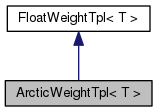ArcticWeightTpl< T > Class Template Reference

`#include <arctic-weight.h>`

Inheritance diagram for ArcticWeightTpl< T >:[legend]
Collaboration diagram for ArcticWeightTpl< T >:[legend]

## Public Types

typedef ArcticWeightTpl< T > ReverseWeight

## Public Member Functions

ArcticWeightTpl ()

ArcticWeightTpl (T f)

ArcticWeightTpl (const ArcticWeightTpl< T > &w)

bool Member () const

ArcticWeightTpl< T > Quantize (float delta=kDelta) const

ArcticWeightTpl< T > Reverse () const

## Static Public Member Functions

static const ArcticWeightTpl< T > Zero ()

static const ArcticWeightTpl< T > One ()

static const std::string & Type ()

static ArcticWeightTpl< T > NoWeight ()

static uint64 Properties ()

## Detailed Description

### template<class T> class fst::ArcticWeightTpl< T >

Definition at line 33 of file arctic-weight.h.

## ◆ ReverseWeight

 typedef ArcticWeightTpl ReverseWeight

Definition at line 37 of file arctic-weight.h.

## ◆ ArcticWeightTpl() [1/3]

 ArcticWeightTpl ( )
inline

Definition at line 39 of file arctic-weight.h.

## ◆ ArcticWeightTpl() [2/3]

 ArcticWeightTpl ( T f )
inline

Definition at line 41 of file arctic-weight.h.

## ◆ ArcticWeightTpl() [3/3]

 ArcticWeightTpl ( const ArcticWeightTpl< T > & w )
inline

Definition at line 43 of file arctic-weight.h.

## ◆ Member()

 bool Member ( ) const
inline

Definition at line 61 of file arctic-weight.h.

61  {
62  // First part fails for IEEE NaN
63  return Value() == Value() && Value() != std::numeric_limits<T>::infinity();
64  }

## ◆ NoWeight()

 static ArcticWeightTpl NoWeight ( )
inlinestatic

Definition at line 57 of file arctic-weight.h.

57  {
58  return ArcticWeightTpl<T>(std::numeric_limits<T>::infinity());
59  }

## ◆ One()

 static const ArcticWeightTpl One ( )
inlinestatic

Definition at line 48 of file arctic-weight.h.

Referenced by kaldi::CreateFactorTransducer().

48  {
49  return ArcticWeightTpl<T>(0.0F); }

## ◆ Properties()

 static uint64 Properties ( )
inlinestatic

Definition at line 77 of file arctic-weight.h.

77  {
78  return kLeftSemiring | kRightSemiring | kCommutative |
79  kPath | kIdempotent;
80  }

## ◆ Quantize()

 ArcticWeightTpl Quantize ( float delta = `kDelta` ) const
inline

Definition at line 66 of file arctic-weight.h.

66  {
67  if (Value() == -std::numeric_limits<T>::infinity() ||
68  Value() == std::numeric_limits<T>::infinity() ||
69  Value() != Value())
70  return *this;
71  else
72  return ArcticWeightTpl<T>(floor(Value()/delta + 0.5F) * delta);
73  }

## ◆ Reverse()

 ArcticWeightTpl Reverse ( ) const
inline

Definition at line 75 of file arctic-weight.h.

75 { return *this; }

## ◆ Type()

 static const std::string& Type ( )
inlinestatic

Definition at line 51 of file arctic-weight.h.

51  {
52  static const std::string type = std::string("arctic") +
54  return type;
55  }

## ◆ Zero()

 static const ArcticWeightTpl Zero ( )
inlinestatic

Definition at line 45 of file arctic-weight.h.

45  {
46  return ArcticWeightTpl<T>(-std::numeric_limits<T>::infinity()); }

The documentation for this class was generated from the following file: